# Explain the concept of stack in C language

Data structure is a collection of data organised in a structured way. It is classified into two types which are linear data structure and non-linear data structure.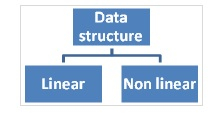Linear data structure − Here, data is organised in a linear fashion.

For example − Arrays, structures, stacks, queues, linked lists.

Non-linear data structure − Here, Data is organised in a hierarchical way.

For example − Trees, graphs, sets, tables.

## Stack in C language

It is a linear data structure, where data is inserted and removed only at one end.

### Operations

• Push – Inserting an element into a stack.
• Pop – Deleting an element from a stack.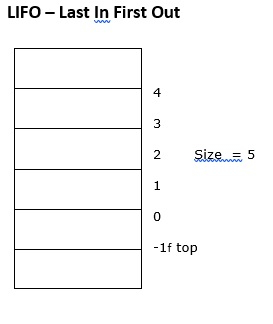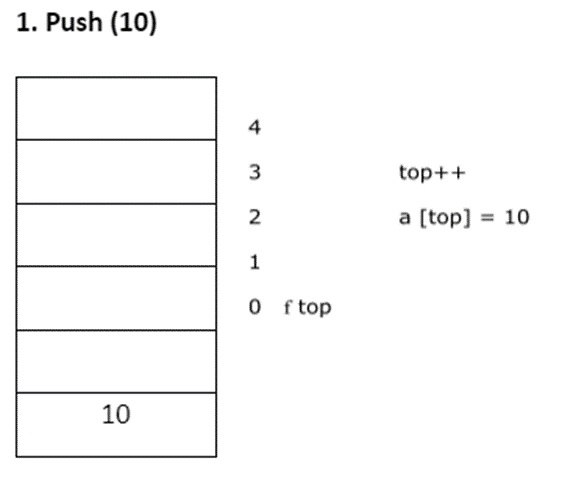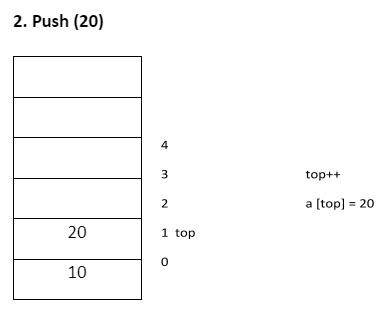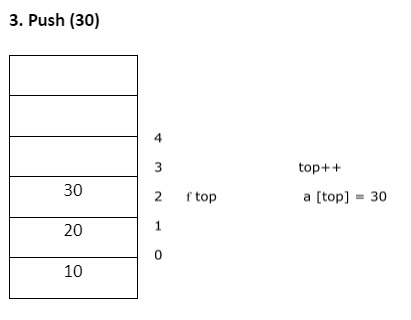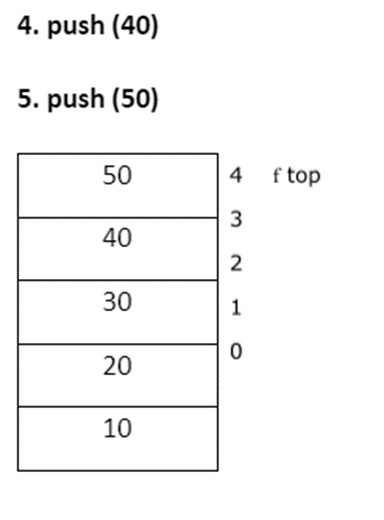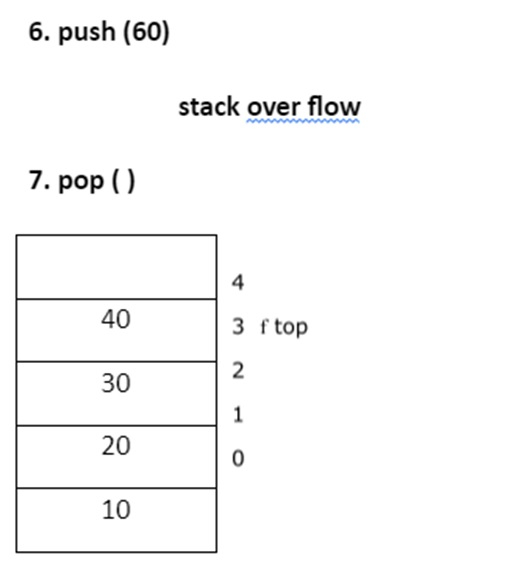Deleted element = 50
Item = a [top]
top --
• pop() ,pop(),pop(), pop()
Deleted element = 40
Deleted element=30
Deleted element=20
Deleted element =10
• Pop ( )

Stack under flow

## Conditions

• Stack over flow − Trying to insert an element into a full stack.

• Stack under flow − Try to delete an element from a stack which is empty.

## Algorithms for Push ( ), Pop ( ) , Display ( )

The respective algorithms are as follows −

### Push ( )

• Check for stack overflow.
if (top = = n-1)
printf("stack over flow”);
• Otherwise, insert an element into the stack.
top ++
a[top] = item

### Pop ( )

• Check for stack underflow.
if ( top = = -1)
printf( "stack under flow”);
• Otherwise, delete the element from the stack.
item = a[top]
top --

### Display ( )

• Check for stack flow.
if (top == -1)
printf ("stack is empty”);
• Otherwise, follow the below mentioned algorithm −
for (i=0; i<top; i++)
printf ("%d”, a[i]);

## Example

Following is the C program for implementation of stack by using the arrays −

#include<stdio.h>
#include <conio.h>
int top = -1, n,a;
main ( ){
int ch;
void pop ( );
void display ( );
clrscr ( );
printf ("enter the size of the stack”);
scanf ("%d”, &n);
printf("stack implementation”);
printf ("1. push ”);
printf ("2. Pop ”);
printf ("3. exit ”);
do{
printf ( "enter ur choice”);
scanf ("%d”, &ch);
switch (ch){
case 1 : push ( );
display ( );
break;
case 2 : push ( );
display ( );
break;
case 3 : exit
}
}while (ch>=1 | | ch<= 3);
getch ( );
}
void push ( ){
int item;
if (top = = n-1)
printf ( "stack over flow”)
else{
printf("enter an element for insertion”)
scanf ("%d”, &item);
top ++;
a[top] = item;
}
}
void pop ( ){
int item;
if (top = = -1);
printf ( "stack under flow”);
else{
item = a[top];
top --;
printf("deleted element = %d”, item);
}
}
void display ( ){
int i;
if (top = = -1)
printf ( "stack is empty”);
else{
printf("contents of the stack are”);
for (i=0; i<top; i++)
printf ("%d \t”, a[i]);
}
}

## Output

When the above program is executed, it produces the following result −

enter the size of the stack = 5 [given by user]
Stack implementation
1. Push 2. Pop 3. exit
Enter ur choice : 1 [given by user]
Enter an element for insertion : 10
Contents of the stack : 10
Enter ur choice : 1
Enter an element for insertion : 2
Contents of the stack : 10 20
Enter ur choice : 2
Deleted element = 20
Contents of the stack are : 10
Enter ur choice : 2
Deleted element : 10
Contents of the stack are : stack is empty
Enter ur choice : 2
Stack underflow.
Enter ur choice : 1
Enter an element for insertion : 30
Contents of the stack are : 30